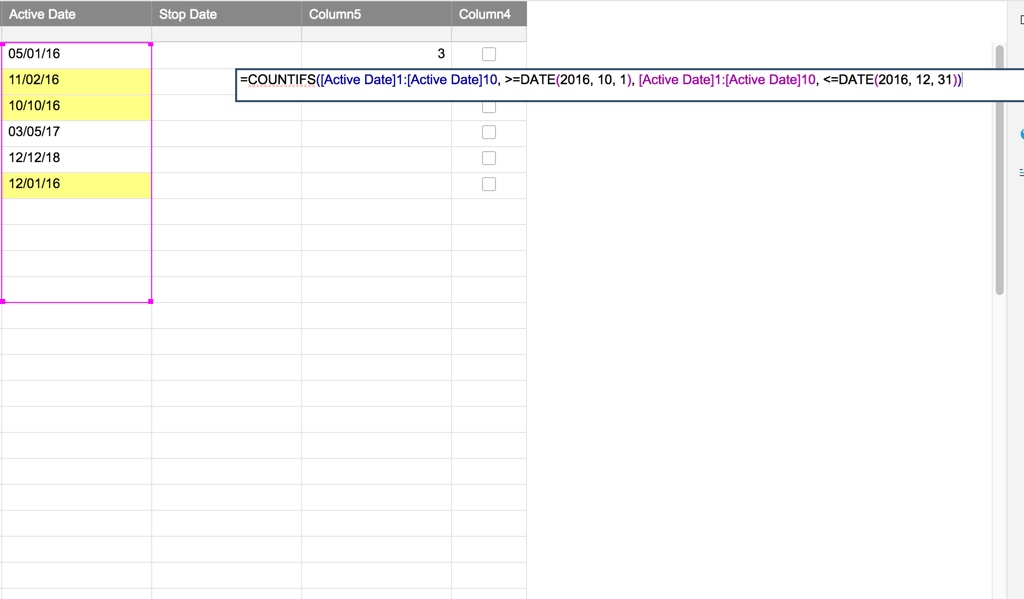# COUNTIFS Formula

Hello Community,

I am encountering difficulty in using COUNTIFS formula to count the items with active dates within the quarter of the year. I tried the below formula in Excel spreadsheet and it worked well. However, the formula doesn't work in Smartsheet.

=COUNTIF(D1:D344, "<10/1/2016")

=COUNTIFS(D1:D344, ">=10/1/2016", D1:D344, "<=12/31/2016")

Formula in Smartsheet:

=COUNTIF([ACTIVE DATE]1:[ACTIVE DATE]344, "<10/01/16")

=COUNTIFS([ACTIVE DATE]1:[ACTIVE DATE]344, ">=10/01/16", [ACTIVE DATE]1:[ACTIVE DATE]344, "<=12/31/16")

I wanted to obtain the count of items activated each quarter of the year.

Someone could help me out please. :-)

Thank you,

Riza

• Have you tried removing the quotes around the dates? Smartsheet will look for EXACTLY what's inside the quotes, so if you <>= symbols aren't next to each date then Smartsheet will assume there is nothing that fits your criteria.

• Yes, I already did. But I received a #INVALID OPERATION error.

• I would recommend using the Date formula instead. https://help.smartsheet.com/function/date

=COUNTIFS([Active Date]1:[Active Date]344, >=DATE(2016, 10, 1), [Active Date]1:[Active Date]344, <=DATE(2016, 12, 31))

• See attached screenshot of working formula.• This is AWESOME! Thank you so much, Mike. I used your provided formula and it works. :-)

Thank you all for taking time to review and to provide recommendations. Truly appreciate it. :-)

Sincerely,

Riza

• Glad I could be of assistance. I always keep this Smartsheet post on hand when working with formulas in Smartsheet.

https://help.smartsheet.com/functions

• They also have a sheet in the templates section called "Smartsheet Formula Examples" that I have found to be pretty handy. It gives examples of the various formulas in action in an actual sheet so you can manipulate data and see what happens.

• Awesome suggestion. I think I'll check that out myself.